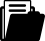Meine Position
i
Ein Tipp vom Eldar Team
Je mehr Sie bestellen, desto grösser Ihr RabattBuch
Buch
Fachbuch
2012

# Intelligent Routines

## Solving Mathematical Analysis with Matlab, Mathcad, Mathematica and Maple

Kollektion

### Intelligent Systems Reference Library

ISBN
EAN
978-3-642-28474-8
9783642284748
Artikel-Nr.
YP8DR77
Sofort lieferbar:
2
Kostenloser Versand
Rabatt
-13.7
%
CHF 291.00
CHF
251.12
Anzahl
1
Maximale
Lieferzeit
5
Arbeitstage
Montag
02.08.2021Beschreibung

Real Analysis is a discipline of intensive study in many institutions of higher education, because it contains useful concepts and fundamental results in the study of mathematics and physics, of the technical disciplines and geometry. This book is the first one of its kind that solves mathematical analysis problems with all four related main software Matlab, Mathcad, Mathematica and Maple. Besides the fundamental theoretical notions, the book contains many exercises, solved both mathematically and by computer, using: Matlab 7.9, Mathcad 14, Mathematica 8 or Maple 15 programming languages. The book is divided into nine chapters, which illustrate the application of the mathematical concepts using the computer. Each chapter presents the fundamental concepts and the elements required to solve the problems contained in that chapter and finishes with some problems left to be solved by the readers. The calculations can be verified by using  a specific software such as Matlab, Mathcad, Mathematica or Maple.

Inhaltsverzeichnis
Sequences and Series of Numbers.- Power Series.- Differentiation Theory of the Functions.- Fundamentals of Field Theory.- Implicit Functions.- Terminology About Integral Calculus.- Equations and Systems of Linear Ordinary Differential Equations.- Line and Double Integral Calculus.- Triple and Surface Integral Calculus.Herausgeber/-innen
Zielgruppe
Research
Leserkritik

From the reviews:

“This book looks at real analysis from successions and power series up to triple and surface integrals, ordinary differential equations are also included. … a very complete text of exercises and problems of classical real analysis from an attractively written perspective. … Given use of software, such as the Matlab, Mathcad, Mathematica and Maple, all well known and used by the academic community … to teach this content, the text may be useful not only for students but also for teachers.” (Mauro Garcia Pupo, Zentralblatt MATH, Vol. 1260, 2013)
Publikation
Deutschland
28.07.2012Sprache
EnglischFormat
Hardcover
582 SeitenVersand
Kostenloser Versand: Schweiz & Liechtenstein
Für den Versand nach Deutschland oder Frankreich werden die Versandgebühren der Schweizerischen Post berechnet. Diese werden Ihnen im Warenkorb für Ihre gesamte Bestellung berechnet.Themen
Unterkategorie
Analysis
Unterkategorie
Informatik
Zurück
Zum Start